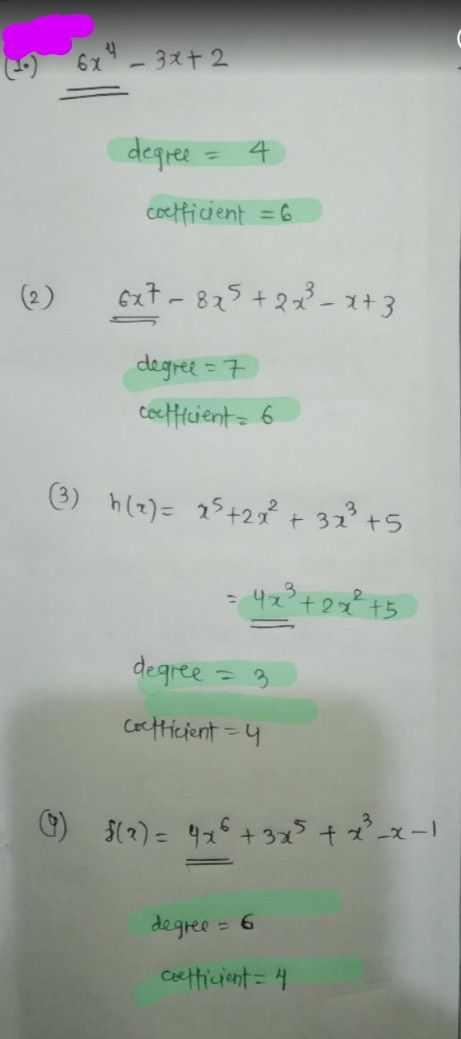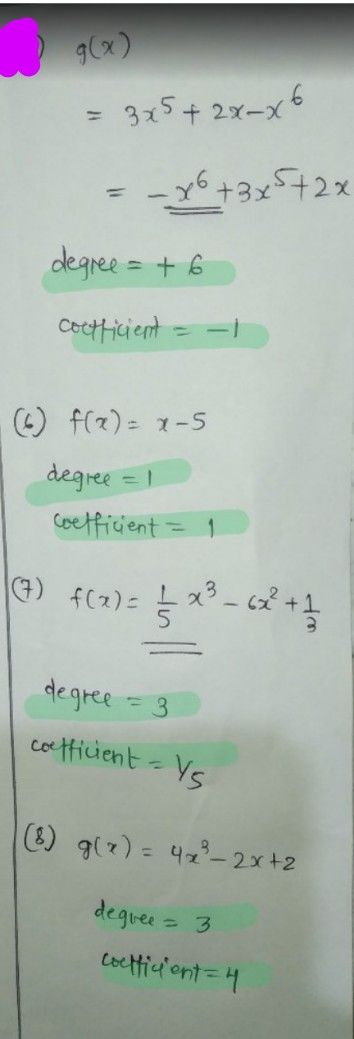Symbol
ProblemHEMATICS 10 . eek $8-Day$ 2 $Obiccav$ To determine the degree of the polynomial and the leading coefficient. Concept Notes The degree of a polynomial is determined by the highest power of its terms. In the polynomial function f(x) = a,x"+ $a$ $n-1x\bar{n} 1+$ $a$ $n-2x^{n-}$ + ...+ ao, where n is a nonnegative integer, and an, an-1, an-2, ..., ao are real numbers, and a,# 0, the degree of the function is n since n is the highest $POwcF$ $Examplcs$ 1) In the polynomial function $f\left(x\right)=2x^{3}$ $6x^{2}+55$ the highest $p0e$ of its terms is 3. Therefore, the degree of the polynomial is 3 and the leading coefficient is 2. 2) The polynomial function $g\left(x\right)=3x^{6}-2x^{4}+x^{2}-x+$ contains the degree of its terms which are 6, $4,2,1$ and 0, respectively. The highest power is 6. Therefore, the degree of the polynomial is and the leading coefficient is 3. $E\times crciscs$ $Direction$ Give the degree of the polynomials and their leading coefficient. 1) $F\left(x\right)=6x^{4}-3x+2$ $P\left(x\right)=6x^{7}-8x^{5}+2x^{3}-x+3$ 56) ) $g\left(x\right)=3x^{5}+2x-x^{6}$ $f\left(x\right)=x-5$ 2) 3) $h\left(x\right)=x^{3}+2x^{2}+3x^{3}+5$ $7\right)f\left(x\right)=\dfrac {1} {5}x^{3}-6x^{2}+\dfrac {1} {3}$ $8\right)g\left(x\right)=4x^{3}-2x+2$ 4) $f\left(x\right)=3x^{5}+x^{3}+4x^{6}-x-1$
10th-13th grade
Algebra
Search count: 115
SolutionQanda teacher - RASHMIKAkindly evaluate ans dearStudent
thank you?Qanda teacher - RASHMIKA
please evaluate ans with gift coins ?